# Arrays

 <  Day Day Up  >

An array is a type that contains a set of variables of the same type. Each element of the array is accessed by its position in the array, called its index (see Figure 1-1). The starting index of an array is always zero.

##### Figure 1-1. A One-Dimensional Array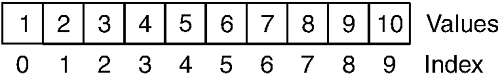The following example creates an array that has ten Integer variables and fills the array with the numbers 1 through 10.

` Dim x(9) As Integer For i As Integer = 0 To 9   x(i) = i + 1 Next i `

Arrays can be multidimensional; that is, they can be indexed by more than one dimension (see Figure 1-2).

##### Figure 1-2. A Multidimensional Array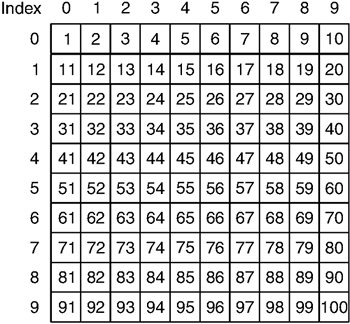The following example creates a two-dimensional array of 100 Integer variables and fills the array with the values 1 through 100.

` Dim x(9, 9) As Integer For i As Integer = 0 To 9   For j As Integer = 0 To 9     x(i, j) = (i * 10) + (j + 1)   Next j Next i `

Array variables can be declared with a preinitialized size by specifying the size as part of the variable declaration. Sizes are always specified in terms of the upper bound of each dimension; in the preceding example, the declaration x(9, 9) As Integer declared an array of 100 elements whose indices ranged from 0 to 9.

Array variables that omit bounds are not initialized to any size and must be created before they can be used. Arrays can be initialized using the ReDim statement.

` Dim x(,) As Integer ReDim x(9,9) For i As Integer = 0 To 9   For j As Integer = 0 To 9     x(i, j) = (i * 10) + (j + 1)   Next j Next i `

Unless the ReDim statement includes the Preserve modifier, an array with values already in it is cleared when it is redimensioned.

Arrays can also be created through an array creation expression. The list that follows the array creation expression either can be empty, indicating the array is not initialized with a set of values, or can contain the values to assign to the array. The following example creates an array of ten Integer values of 0 (the default value of Integer ) and assigns it to the variable x . Then it creates an array of ten Integer values with the values 1 through 10 and assigns it to the variable y .

` Dim x(), y() As Integer x = New Integer(9) {} y = New Integer() { 1, 2, 3, 4, 5, 6, 7, 8, 9, 10 } `

The element type of an array can be an array type itself, in which case the element of the outer array is an inner array (see Figure 1-3), which must be created before it can be used. This is in contrast to a multidimensional array (see Figure 1-2), where all elements always exist.

##### Figure 1-3. An Array of Arrays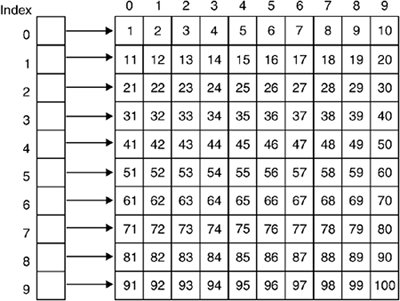In the following example, the variable x contains an array of ten one-dimensional arrays of Integer . Each element of the array must first be initialized with another array before it can be used. In contrast, the variable y contains a two-dimensional array of 100 Integer values. No extra initialization is required.

` Dim x()() As Integer Dim y(,) As Integer x = New Integer(9)() {} y = New Integer(9,9) {} For i As Integer = 0 To 9   x(i) = New Integer(9) {}   For j As Integer = 0 To 9     x(i)(j) = (i * 10) + (j + 1)     y(i, j) = (i * 10) + (j + 1)   Next j Next i `

Arrays of arrays can be jagged ”that is, their dimensions can be uneven (see Figure 1-4) ”while multidimensional arrays are always rectangular.

##### Figure 1-4. A Jagged Array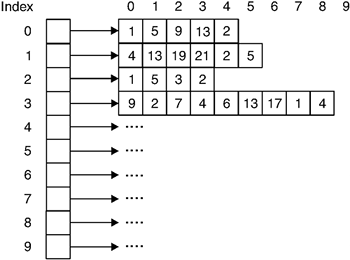<  Day Day Up  >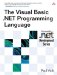The Visual Basic .NET Programming Language
ISBN: 0321169514
EAN: 2147483647
Year: 2004
Pages: 173
Authors: Paul Vick

Similar book on Amazon